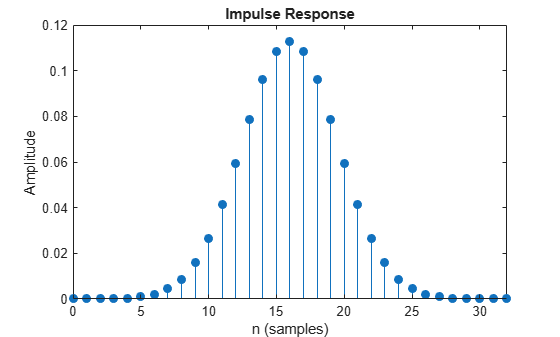Main Content

# gaussdesign

Gaussian FIR pulse-shaping filter design

## Syntax

``h = gaussdesign(bt,span,sps)``

## Description

example

````h = gaussdesign(bt,span,sps)` designs a lowpass FIR Gaussian pulse-shaping filter and returns a vector, `h`, of filter coefficients. The filter is truncated to `span` symbols, and each symbol period contains `sps` samples. The order of the filter, `sps*span`, must be even.```

## Examples

collapse all

Specify that the modulation used to transmit the bits is a Gaussian minimum-shift keying (GMSK) pulse. This pulse has a 3-dB bandwidth equal to 0.3 of the bit rate. Truncate the filter to 4 symbols and represent each symbol with 8 samples.

```bt = 0.3; span = 4; sps = 8; h = gaussdesign(bt,span,sps); fvtool(h,'impulse')```## Input Arguments

collapse all

Product of the 3-dB one-sided bandwidth, in hertz, and the symbol time, in seconds. Specify this value as a positive real scalar. Smaller values of `bt` produce larger pulse widths.

Data Types: `double` | `single`

Number of symbols, specified as a positive integer scalar.

Data Types: `double` | `single`

Number of samples per symbol period (oversampling factor), specified as a positive integer scalar.

Data Types: `double` | `single`

## Output Arguments

collapse all

FIR coefficients of the Gaussian pulse-shaping filter, returned as a row vector. The coefficients are normalized so that the nominal passband gain is always 1.

Data Types: `double` | `single`

## Algorithms

The impulse response of the Gaussian filter is given by

`$h\left(t\right)=\frac{\mathrm{exp}\left(\frac{-{t}^{2}}{2{\delta }^{2}}\right)}{\sqrt{2\pi }\cdot \delta }$`

where

`$\delta =\frac{\sqrt{\mathrm{log}2}}{2\pi BT}.$`

BT is the bandwidth-symbol time product specified in `bt`, where B is the 3-dB bandwidth of the filter and T is the symbol time. The number of symbols between the start and end of the impulse (`span`) and the number of samples per symbol (`sps`) determine the length of the impulse response: $span×sps+1.$

For more information, see FIR Gaussian Pulse-Shaping Filter Design.

 Krishnapura, N., S. Pavan, C. Mathiazhagan, and B. Ramamurthi. “A baseband pulse shaping filter for Gaussian minimum shift keying.” Proceedings of the 1998 IEEE International Symposium on Circuits and Systems. Vol. 1, 1998, pp. 249–252.

 Rappaport, Theodore S. Wireless Communications: Principles and Practice. 2nd Ed. Upper Saddle River, NJ: Prentice Hall, 2002.

## Support

#### Deep Learning for Signal Processing with MATLAB

Download white paper